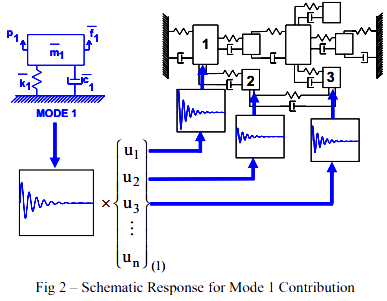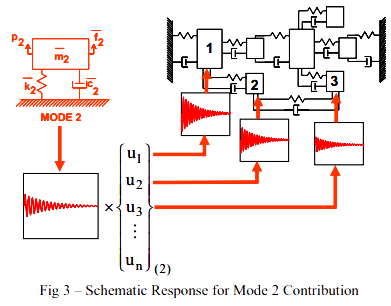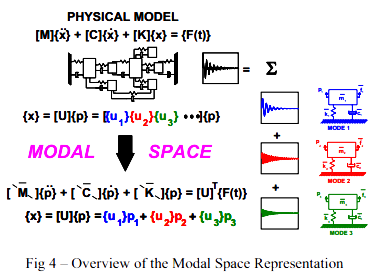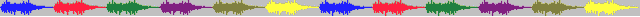# 75.模态空间10.06-模态空间中单自由度是如何跟物理响应联系起来的？我依然感到困惑。

### 模态空间 – 在我们自己的小世界中     Pete Avitabile(著) KSI科尚仪器 董书伟（译）#### 这需要讨论。

2014年04月12日 发布 ver1.0

$\left [ M \right ]\left \{ \ddot{x} \right \}+\left [ C \right ]\left \{ \dot{x} \right \}+\left [ K \right ]\left \{ x \right \}=\left \{ F\left ( t \right ) \right \}$

$\left \{ x \right \}=\left [ U \right ]\left \{ p \right \}=\left \{ u_1 \right \}p_{1}+\left \{ u_2 \right \}p_{2}+\left \{ u_3 \right \}p_{3}+\,\cdots$

$\quad \left [ U \right ]=\begin{bmatrix} \left \{ u_1 \right \} &\left \{ u_2 \right \} &\left \{ u_3 \right \} & \cdots \end{bmatrix}$

$\begin{bmatrix} \bar{m}_1 & & \\ &\bar{m}_2 & \\ & &\setminus \end{bmatrix}\begin{Bmatrix} \ddot{p}_1\\ \ddot{p}_2\\ \vdots \end{Bmatrix}+\begin{bmatrix} \bar{c}_1 & & \\ &\bar{c}_2 & \\ & &\setminus \end{bmatrix}\begin{Bmatrix} \dot{p}_1\\ \dot{p}_2\\ \vdots \end{Bmatrix}+ \begin{bmatrix} \bar{k}_1 & & \\ &\bar{k}_2 & \\ & &\setminus \end{bmatrix}\begin{Bmatrix} {p}_1\\ {p}_2\\ \vdots \end{Bmatrix}=\begin{Bmatrix} \left \{ u_1 \right \}^{T}\left \{ F \right \}\\ \left \{ u_2 \right \}^{T}\left \{ F \right \}\\ \vdots \end{Bmatrix}$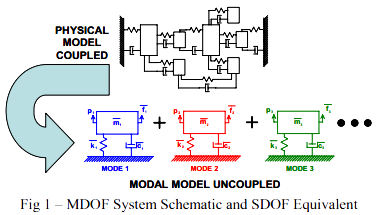$\bar{m}_{i}\ddot{p}_{i}+\bar{c}_{i}\dot{p}_{i}+\bar{k}_{i}{p}_{i}=\left \{ u_i \right \}^{T}\left \{ F \right \}=\bar{f}_i$